# Test: Stability

Test Description

## 15 Questions MCQ Test GATE Electrical Engineering (EE) 2023 Mock Test Series | Test: Stability

Test: Stability for Electronics and Communication Engineering (ECE) 2023 is part of GATE Electrical Engineering (EE) 2023 Mock Test Series preparation. The Test: Stability questions and answers have been prepared according to the Electronics and Communication Engineering (ECE) exam syllabus.The Test: Stability MCQs are made for Electronics and Communication Engineering (ECE) 2023 Exam. Find important definitions, questions, notes, meanings, examples, exercises, MCQs and online tests for Test: Stability below.
Solutions of Test: Stability questions in English are available as part of our GATE Electrical Engineering (EE) 2023 Mock Test Series for Electronics and Communication Engineering (ECE) & Test: Stability solutions in Hindi for GATE Electrical Engineering (EE) 2023 Mock Test Series course. Download more important topics, notes, lectures and mock test series for Electronics and Communication Engineering (ECE) Exam by signing up for free. Attempt Test: Stability | 15 questions in 45 minutes | Mock test for Electronics and Communication Engineering (ECE) preparation | Free important questions MCQ to study GATE Electrical Engineering (EE) 2023 Mock Test Series for Electronics and Communication Engineering (ECE) Exam | Download free PDF with solutions
 1 Crore+ students have signed up on EduRev. Have you?
Test: Stability - Question 1

### The forward-path transfer function of a ufb system is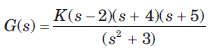For system to be stable, the range of K is

Detailed Solution for Test: Stability - Question 1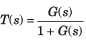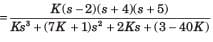Routh table is as shown in fig. S.6.211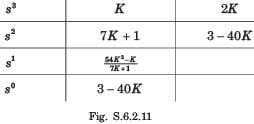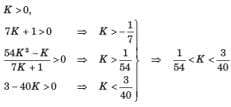Test: Stability - Question 2

### Which among these is a classification of power system stability?

Test: Stability - Question 3

### The open-loop transfer function of a ufb system is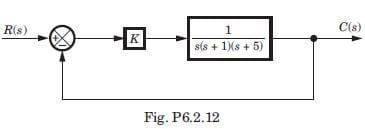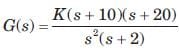The closed loop system will be stable if the value of K is

Detailed Solution for Test: Stability - Question 3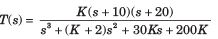Routh table is as shown in fig.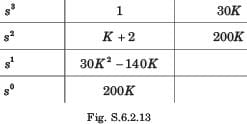200K > 0 → K > 0, 30K2 - 140K > 0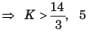satisfy this condition.

Test: Stability - Question 4

The closed loop transfer function for this system is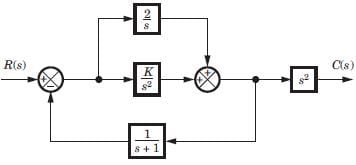Detailed Solution for Test: Stability - Question 4

First combine the parallel loop K/s2
and 2/s giving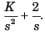Then apply feedback formula with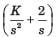and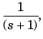and then multiply with s2.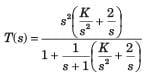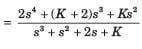Test: Stability - Question 5

The poles location for this system is shown in fig.The value of K is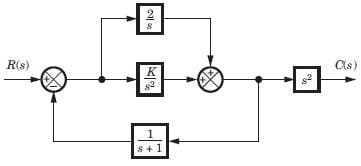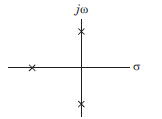Detailed Solution for Test: Stability - Question 5

Denominator = s3 + s2 + 2s + K Routh table is as shown in fig.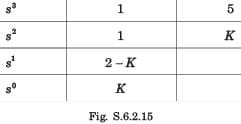Row of zeros when K = 2,
s2 + 2 = 0, ⇒ s = -1, j√2, - j√2

Test: Stability - Question 6

The forward path transfer of ufb system is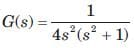The system is

Test: Stability - Question 7

The forward-path transfer function of a ufb system is T(s) =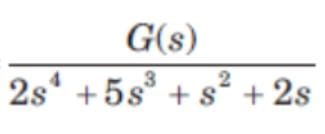The system is

Detailed Solution for Test: Stability - Question 7

Closed loop transfer function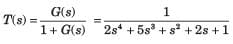Routh table is as shown in fig. S.6.2.28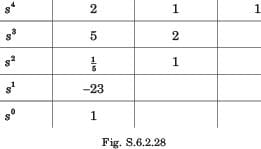2 RHP poles so unstable.

Test: Stability - Question 8

The open loop transfer function of a system is as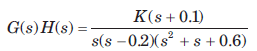The range of K for stable system will be

Detailed Solution for Test: Stability - Question 8

The characteristic equation is 1 + G(s)H(s) = 0

⇒ s(s - 0.2)(s2 + s + 0.6)+K(s + 0.1) = 0
s4 +0.8 s3 +0.4s2 +(K - 0.12)s +0.1K = 0
Routh table is as shown in fig. S.62.29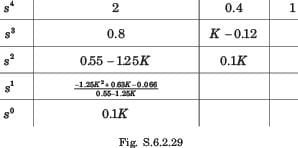K > 0, 055 -125K > 0 ⇒ K < 0.44 -125K2 +0.63K -0066 >0
(K - 0.149)(K - 0355) < 0, 0.149 < K < 0.355

Test: Stability - Question 9

The open-loop transfer function of a ufb control system is given by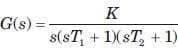For the system to be stable the range of K is

Detailed Solution for Test: Stability - Question 9

Characteristic equation

s(sT1 + 1)(sT2 +1) + K = 0
T1T2s3 + (T1 + T2)s2 + s + K = 0
Routh table is as shown in fig S.6.2.30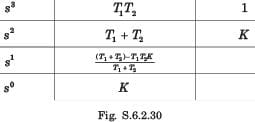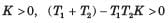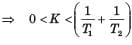Test: Stability - Question 10

If the roots of the have negative real parts, then the response is ____________

Detailed Solution for Test: Stability - Question 10

If the roots of the have negative real parts then the response is bounded and eventually decreases to zero.

Test: Stability - Question 11

The closed loop transfer function of a system is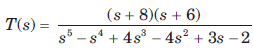The number of poles in RHP and in LHP are

Detailed Solution for Test: Stability - Question 11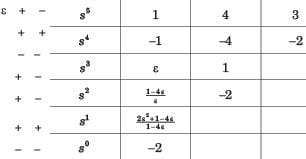3 RHP, 2 LHP poles.

Test: Stability - Question 12

The closed loop transfer function of a system is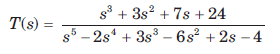The number of poles in LHP, in RHP, and on jω - axis are

Detailed Solution for Test: Stability - Question 12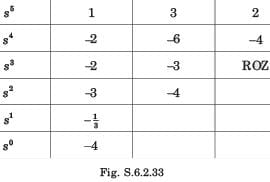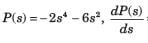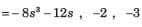No sign change exist from the s4 row down to the s0 row.
Thus, the even polynomial does not have RHP poles. Therefore because of symmetry all four poles must be on jw -axis.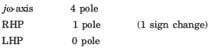Test: Stability - Question 13

For the system shown in fig. the number of poles on RHP, LHP, and imaginary axis are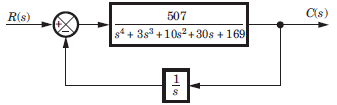Detailed Solution for Test: Stability - Question 13

Closed loop transfer function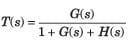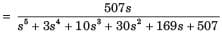Routh table is as shown in fig. S.6.2.34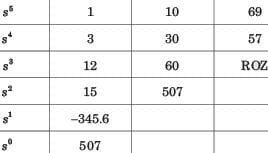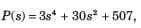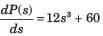From s4 row down to s0 there is one sign change. So LHP–1 + 1= 2 pole. RHP–1 pole, jw - axis - 2 pole.

Test: Stability - Question 14

If a system is given unbounded input then the system is:

Detailed Solution for Test: Stability - Question 14

If the system is given with the unbounded input then nothing can be clarified for the stability of the system.

Test: Stability - Question 15

For the open loop system of fig. location of poles on RHP, LHP, and an jω - axis are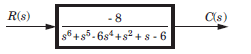Detailed Solution for Test: Stability - Question 15

Routh table is as shown in fig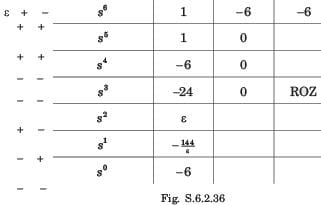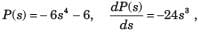Them is two sign change from the s4 mw down to the s° row. So two roots are on RHS. Because of symmetry rest two roots must be in LHP. From s6 to s4 there is 1 sign change so 1 on RHP and 1 on LHP.
Total LHP 3 root, RHP 3 root.

## GATE Electrical Engineering (EE) 2023 Mock Test Series

22 docs|274 tests
 Use Code STAYHOME200 and get INR 200 additional OFF Use Coupon Code